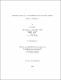## Most likely path to the shortfall risk under the optimal hedging strategy2012
##### Publisher
University of Alabama Libraries
##### Abstract

In this dissertation, we search for the most likely path to the shortfall risk in hedging a long-term supply commitment with short-term futures contracts, which leads to a class of optimization problems. Motivated by a simple model initially discussed in Culp and Miller , Mello and Parsons  and Glasserman , the optimal hedging strategy provided by Larcher and Leobacher , and the simple discussion about the most likely path by Glasserman , we studied the following optimization problem: $$\min_{\phi \in \mathcal{A}{x}} \frac{1}{2} \int_0^1 \left[ \dot{\phi}(t) \right]^2 dt$$ where $$\mathcal{A}{x}=\left{ \phi: \sigma \int_0^t [G(s)+s-t] dW_s \leq -x, textrm{for some tin[0,1]} \right}.$$ We showed the general form of the most likely path under a hedging strategy. We obtained most likely paths under the optimal-fraction hedging strategy provided by Glasserman  and found that the most likely path is not unique. The main work in this dissertation is to search for the most likely path to the shortfall risk under the optimal hedging strategy provided by Larcher and Leobacher .

##### Description
Electronic Thesis or Dissertation
Mathematics